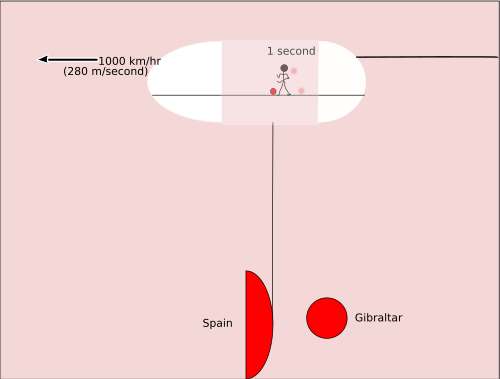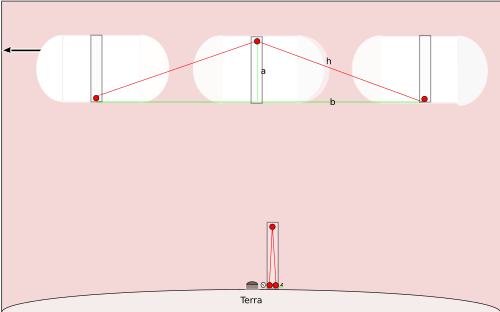# Light clock facts for kids

Kids Encyclopedia Facts

The light clock is a simple way of showing a basic feature of Special relativity. A clock is designed to work by bouncing a flash of light off a distant mirror and using its return to trigger another flash of light, meanwhile counting how many flashes have occurred along the way. It is easy to show that people on Earth watching a spaceship fly overhead with such a clock would see it ticking relatively slowly. This effect is called time dilation.

Before we study the light clock, consider another kind of relativity. Imagine that someone is dribbling a basketball in the hold of a large cargo jet. The basketball player is moving in the same direction as the jet airplane. The other people in the plane see him moving a meter or two as he makes one dribble. Between the time the ball bounces the first time and the ball bounces the second time, about one second has passed. But when the first bounce occurred the basketball was over Gibraltar, and when the second bounce occurred the basketball was over the water nearer to Spain. So the basketball has moved 280 meters with respect to Earth.Now consider a somewhat similar question of relative motion. This time we are going to consider what people looking at stars from the North Pole see when a very fast spaceship flies over them. We can use algebra and the Pythagorean theorem to calculate how much time slows on the spaceship. The only other thing we need is the equation that relates distance, d, rate or speed of travel, r, and time, t. The equation is:

d = rt

The speed of light is constant, so we will apply this value to two problems. We will call the speed of light c, as this is the letter scientists usually use to name it.A clock is made by putting a light output at the bottom of a long pole, a mirror on top of the pole, and an electronic light detector down at the bottom of the pole. The clock is started by briefly closing a switch that sends one blink of light from the bottom of the pole to the top of the pole where it is reflected back to the bottom of the pole. When the light detector at the bottom of the pole sees the blink of light it does two things. It adds one to the counter that is attached to it, and it fires another blink of light up to the mirror. When that blink of light gets back to the bottom, the count changes to two, and another blink of light is set off. Since light travels very rapidly (300,000 kilometers/second), for each second measured by an ordinary clock the light clock will "tick" a very large number of times.

To make the math easier, we will say that the pole is one half kilometer long. So if we are standing by the light clock that is built next to the big telescope at the North Pole, we will see that light travels one kilometer for each "tick" of the light clock. Since distance traveled, d, equals speed multiplied by time, and the speed involved is c, we have the equation:

d = ct

and we can solve this equation for t to learn how long in seconds each "tick" is.

1 km = 300,000 km/second * t seconds

t seconds = 1 km/300,000 (km/second) = 1/300,000 seconds = 0.00000333…3 seconds

In other words, each "tick" of the light clock will take 0.00000333…3 seconds.

If a spaceship were flying on a straight line passing over the North Pole at a large fraction of the speed of light, and it had a similar clock, people watching its passage would see that the mirror on top of the pole had moved from directly over the emitting light, so the light would travel along the line marked h in the diagram, and then it would follow the other hypotenuse back down to the base of the pole -- which would by now have moved some distance since the spaceship is moving so fast. We can figure out how much time one tick would take according to the people on Earth. We know that the spaceship's pole has a length of a since it is the same kind of clock as people are using at the North Pole. We want to figure out t' , the time it takes to make one tick of the clock on the spaceship.

We know that the spaceship will travel 1/2 r t' while the blink of light is heading up toward the mirror, and another 1/2 r t' while the blink of light is heading down toward the base of the pole. So that calculation gives us the length of line b in the chart. We know a, so we can figure out h by the Pythagorean theorem:

h = √(a2 + (rt' /2)2)

So the total distance the light travels is 2 h or d = 2 √(a2 + (rt' /2)2)

We also know that the speed of light, c, is constant. No matter who measures it, it turns out to be the same speed. So we can use that fact to get another way of calculating how long it takes for the flash of light to go from the base to the top of the pole and back again:

t' = d/c

In other words, d = c t' .

So we can write

c t' = 2 √(a2 + (rt' /2)2)

or

1/2 c t' = √(a2 + (rt' /2)2)

To solve the above equation, we will need to:

1. Square both sides
2. Divide both sides by t' 2
3. Multiply both sides by 4
4. Divide both sides by c2
5. Simplify c2 / c2
6. Subtract r2/c2 from both sides
7. Take the square root of both sides
8. Multiply both sides by t'
9. Divide both sides by √(1-r2/c2)

Solving the above equation we find that:

t' = 2a/(c√(1-r2/c2)

The time between ticks on the clock at the North Pole is 2a/c, so we can then write:

t' = t/√(1-r2/c2)

If t = 1 second, then if the spaceship is traveling at one half the speed of light, t' = 1.1547 sec.

Experiment with various speeds of travel at: http://www.1728.org/reltivty.htm

## Images for kidsLight clock Facts for Kids. Kiddle Encyclopedia.